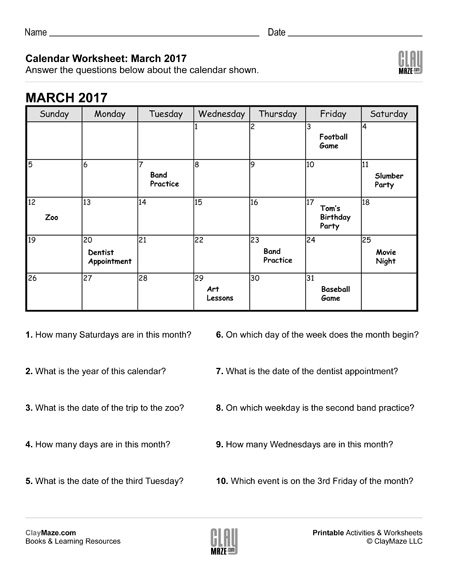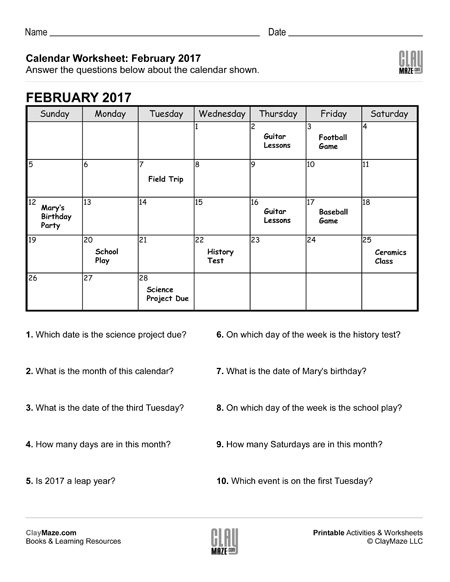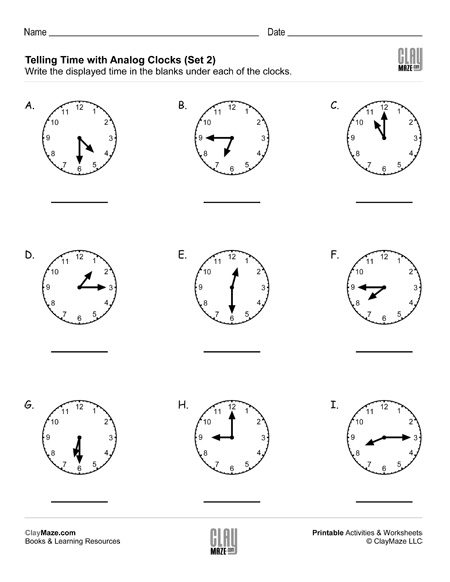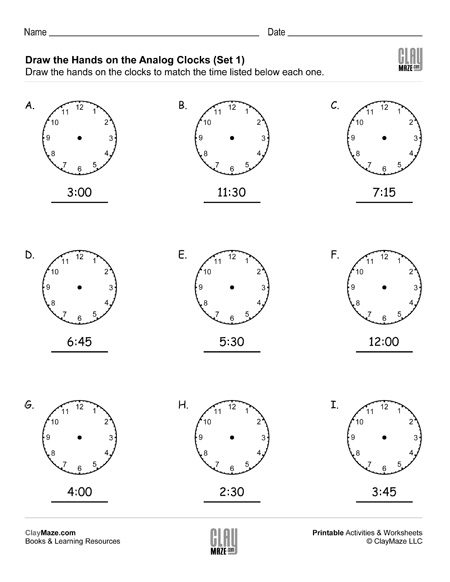## Topic: Date & Time

Free Printable Worksheets on Date and Time – Calendars and Clocks

## Blank CalendarDownload our blank calendar template for children to make their own calendars. It can also be used as a weekly schedule tool....

## Reading a Calendar Worksheet – AThis worksheet displays a calendar month with the year, along with events that are to take place on different dates. The student is asked questions about the calendar regarding dates, days of the...

## Reading a Calendar Worksheet – BThe worksheet has a calendar filled out with a few events on different dates. There are questions below the calendar asking about the calendar on the worksheet....

## Reading a Calendar Worksheet – CThis worksheet is for practice reading a calendar. Below the calendar are questions about the calendar, weekdays, dates and the events displayed....

## Reading a Calendar Worksheet – DThis worksheet features a calendar with events posted on different dates. Following that are questions about the calendar, dates, weekdays and events listed....

## Telling Time – Read the Analog Clocks (Set 1)This worksheet is good practice for telling time with analog clocks. There are 9 clocks and the student is asked to figure out the time displayed on the clocks and then write the time for each one....

## Telling Time – Read the Analog Clocks (Set 2)Download this worksheet for lots of practice with telling time on analog clocks. The worksheet features 9 clocks and asks the student to write the correct time under each one....

## Telling Time – Read the Analog Clocks (Set 3)This is an analog clock worksheet for practice telling time. Have the student write the correct time in the blank for each clock. There are 9 clocks....

## Telling Time – Read the Analog Clocks (Set 4)Print this free worksheet on telling time with analog clocks. There are 9 clocks with different times. The student is asked to write the correct time in the blanks below the clocks....

## Draw the Hands on the Analog Clocks (Set 1)Draw the hands on the analog clocks to match the time given below each one. Remember the hour hand moves slowly as the minute hands moves around the clock. There are 9 clocks per worksheet....

## Draw the Hands on the Analog Clocks (Set 2)Use this free worksheet for practice telling time with analog clocks. On this one, the student is asked to draw the hands on the clocks. Make sure they remember the hour hands moves slowly as the...

## Draw the Hands on the Analog Clocks (Set 3)This worksheet is great practice with analog clocks. Have the student draw the hands on the clock to match the time given for each one. Make sure the hour hands are drawn correctly taking their...

## Draw the Hands on the Analog Clocks (Set 4)Download this free worksheet for analog clock practice. Draw the hands on each of the clocks to match the times given. Don’t forget the movement of the hour hand as the minute hand goes around the ...

## Time Word Problems: Analog ClocksThese sets of worksheets are great for practice telling time with analog clocks and for understanding how to add and subtract with different time intervals. On these worksheets, the student is aske...